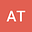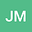Higher order stable schemes for stochastic convection-reaction-diffusion equations driven by additive Wiener noise
••• Antoine Tambue,
• Jean Daniel Mukam
Antoine Tambue
Western Norway University of Applied Sciences

Corresponding Author:antonio@aims.ac.za

Author ProfileJean Daniel Mukam
Technische Universitat Chemnitz
Author Profile## Abstract

In this paper, we investigate the numerical approximation of stochastic convection-reaction-diffusion equations using two explicit exponential integrators. The stochastic partial differential equation (SPDE) is driven by additive Wiener process. The approximation in space is done via a combination of the standard finite element method and the Galerkin projection method. Using the linear functional of the noise, we construct two accelerated numerical methods, which achieve higher convergence orders. In particular, we achieve convergence rates approximately $1$ for trace class noise and $\frac{1}{2}$ for space-time white noise. These convergences orders are obtained under less regularities assumptions on the nonlinear drift function than those used in the literature for stochastic reaction-diffusion equations. Numerical experiments to illustrate our theoretical results are provided
26 Jul 2020Submitted to Mathematical Methods in the Applied Sciences
30 Jul 2020Submission Checks Completed
30 Jul 2020Assigned to Editor
01 Aug 2020Reviewer(s) Assigned
15 Dec 2020Review(s) Completed, Editorial Evaluation Pending
08 Jan 2021Editorial Decision: Revise Minor